# Load countless 3D objects in Three.js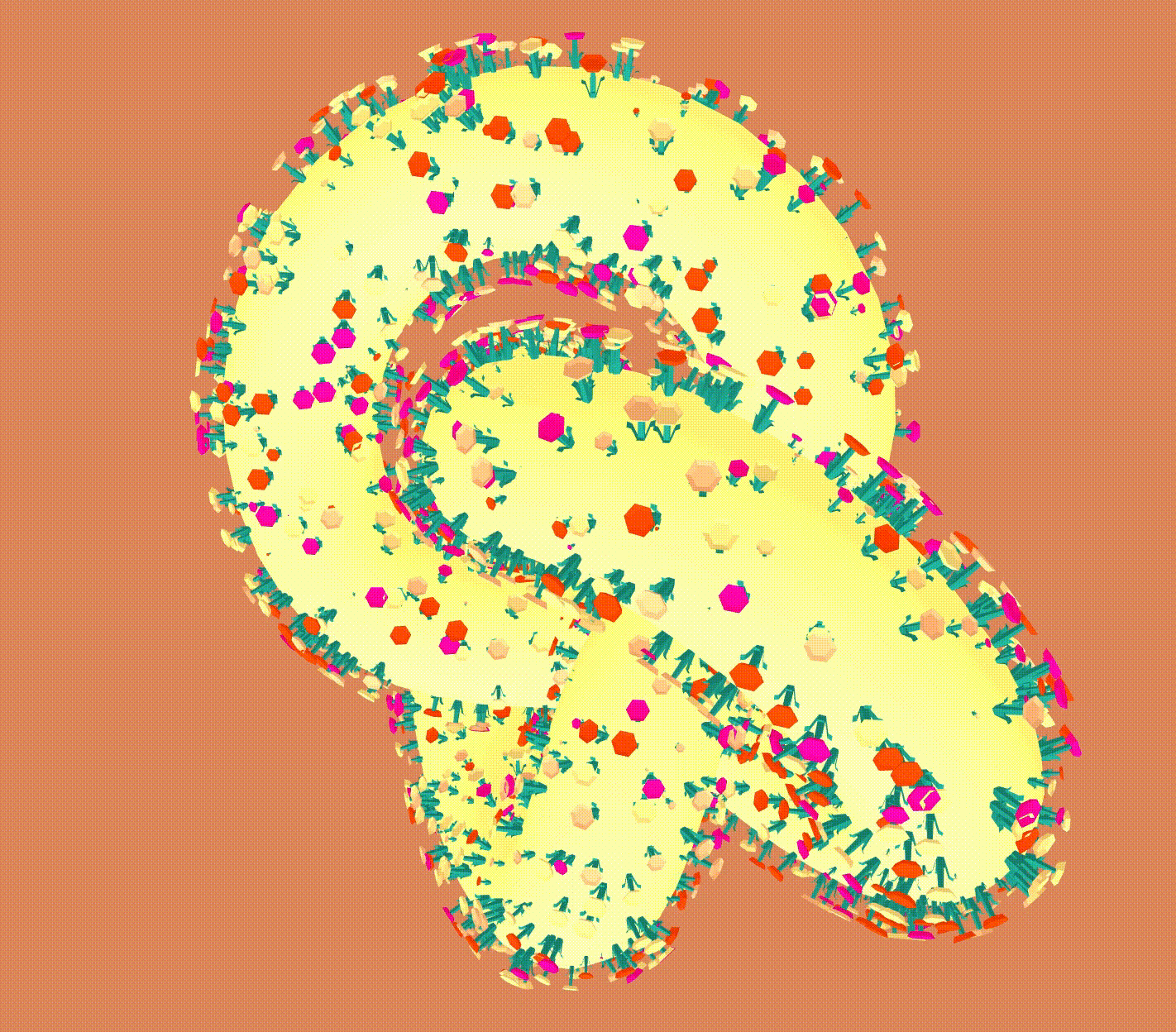## Today’s Example

I have been practising to quickly read an example code in three.js repository. The example that I have scanned is to load numerous objects with infinite repetitive animation on a particular geometric object.

#### Logic

The logic to animate is broadly the following procedure.

1. Set up Scene, Camera, Mesh placed on a scene
3. set up flower objects using model
4. render
* reset and replace the flower if it has been dead
* update scale

In this article, I will pick up how the flower object is regenerated at constant.

## Get Object from Object Name

There are many flowers when launching the example code. Where do they come from? Do we have to load the objects, respectively?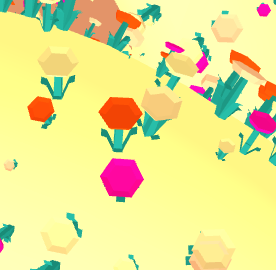We do not have to load thousands of models at a time but do it once. What we consider about the model is the name of the flower object. To be scaling it up in every frame, we have to manipulate each object consisted of a stem and a blossom. The names are already assigned in the ready-made model in Three.js repository. You can find them on Blender below.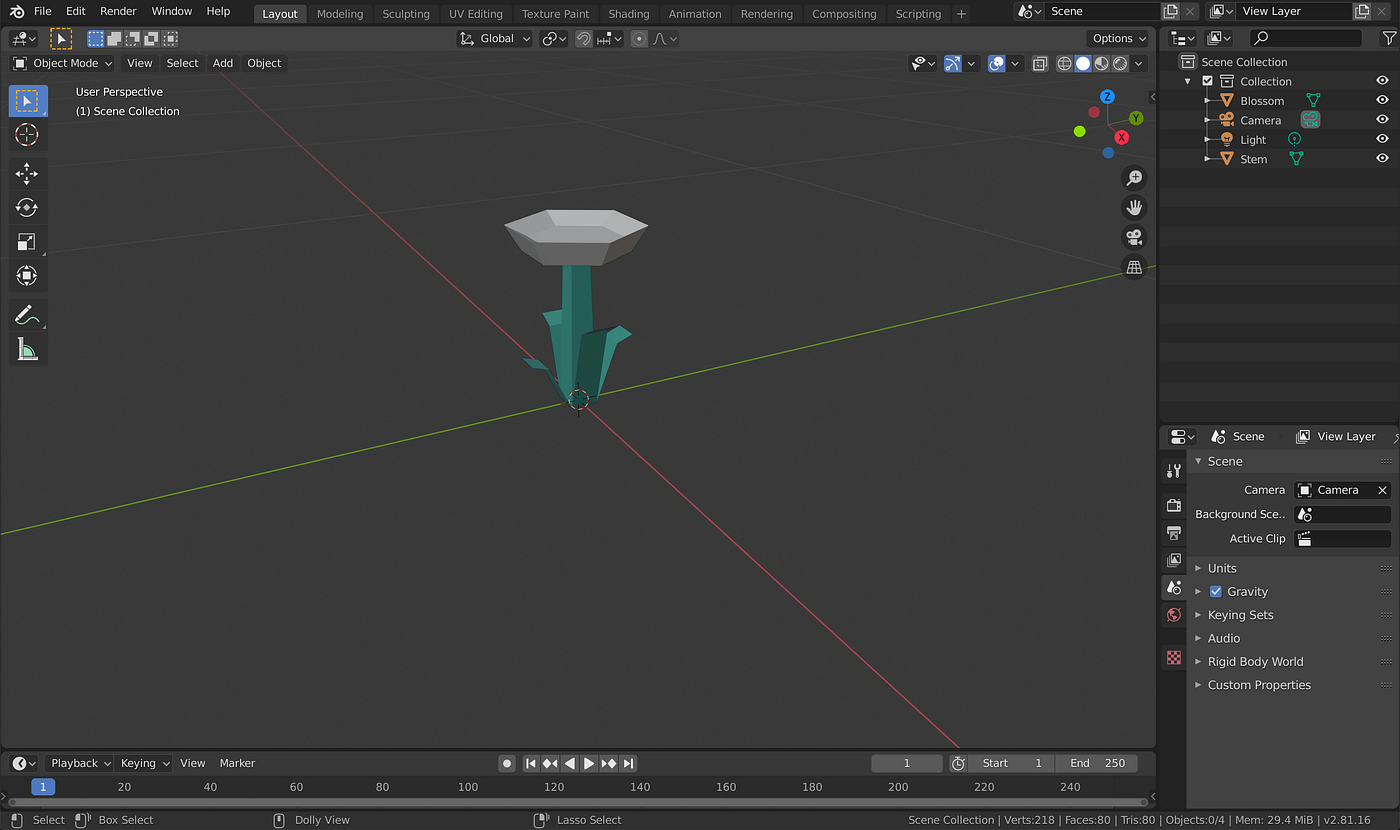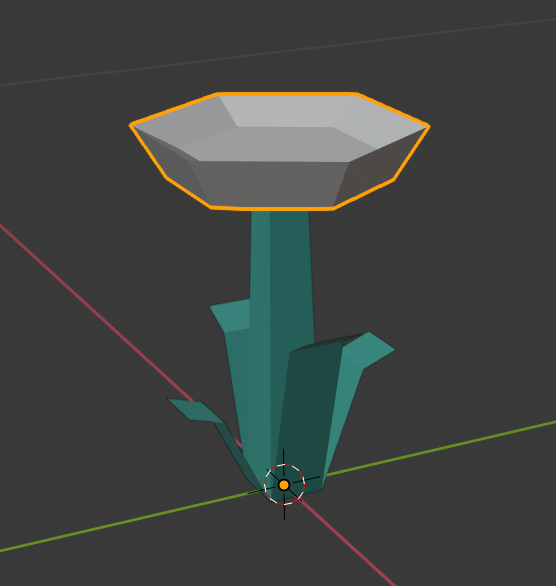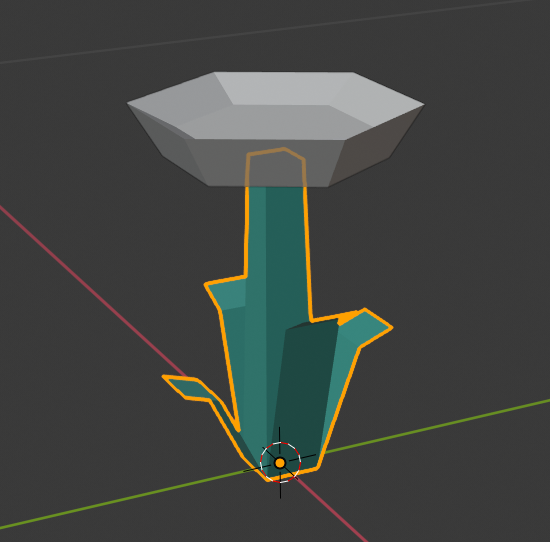Then we can create geometries using the names in Three.js. The key method is THREE.Object3D.getObjectByName. These names in the model are searchable through with the function at the side of Three.js.

var loader = new GLTFLoader();loader.load('./models/gltf/Flower/Flower.glb', function (gltf) {
var _stemMesh = gltf.scene.getObjectByName('Stem');var _blossomMesh =
gltf.scene.getObjectByName('Blossom');
stemGeometry = new THREE.InstancedBufferGeometry();
blossomGeometry = new THREE.InstancedBufferGeometry();
THREE.BufferGeometry.prototype.copy.call(stemGeometry, _stemMesh.geometry);
THREE.BufferGeometry.prototype.copy.call(blossomGeometry, _blossomMesh.geometry);
}

After creating a couple of a stem and a blossom geometries, we can prepare a large number of flower Mesh objects. Three.js supports to create them by using THREE.InstancedMesh.

stemMesh = new THREE.InstancedMesh(stemGeometry, stemMaterial, count);
blossomMesh = new THREE.InstancedMesh(blossomGeometry, blossomMaterial, count);

## Scaling by Generation

There are a few steps to scale an object up in every frame.

Firstly, set up the initial scale and age that is pointing to how the size is mounting up. The scale is scaling at the curve of easeOutCubic, and the age is growing up by 0.005 in every frame.

// Source: https://gist.github.com/gre/1650294
var easeOutCubic = (t) => (--t) * t * t + 1;
// disappear quickly. More of the particle's lifetime is spent around full scale.
var scaleCurve = (t) => Math.abs(easeOutCubic((t > 0.5 ? 1 - t : t) * 2))
for (var i = 0; i < count; i++) {
ages[i] = Math.random();
scales[i] = scaleCurve(ages[i]);
}

The point of highlight in the scaling is how the proportion between the previous size and the current one is. I was struggling to figure out the logic. In the update function that is called on every frame, the age increased at first. Next, preserve the previous scale from the array of size, then insert the current size into i

ages[i] += 0.005;
var prevScale = scales[i];
scales[i] = scaleCurve(ages[i]);

Finally, calculate the ratio of the current scale to the previous one. The relative scale rate will be assigned.

// NOTE: relative ratio by previous scale and current value.
// If previous is 0.5 and current is also 0.5, it means nothing to do.
// If previous is 0.5 and current is 0.6, it relatively increases at 1.2
_scale.set(scales[i] / prevScale, scales[i] / prevScale, scales[i] / prevScale);

The age goes up to the end of limit, then die. That is to say; it will be refreshed in another position at the initial scale. Once the age count reaches 1.0, it means to be dead for the flower, then reborn at the another location and size.

## Conclusion

I have learned that when creating countless objects, I had better use THREE.InstancedMesh. Keep in mind to set object name in model beforehand. Scaling algorithm in this demo would be a bit useful in the future. I hope this article is informative for some developers.

#### You may also like